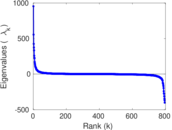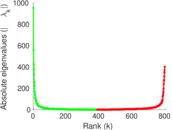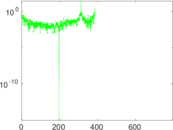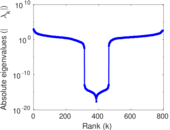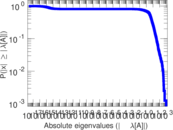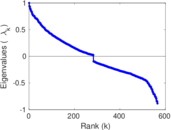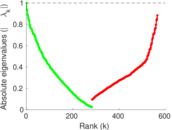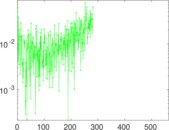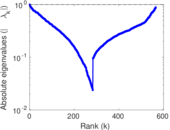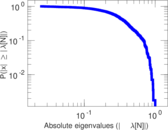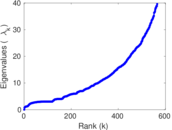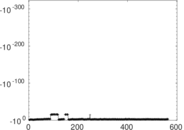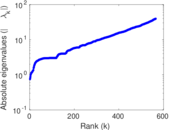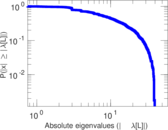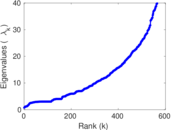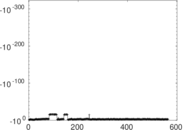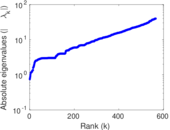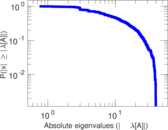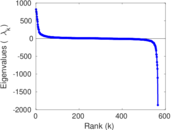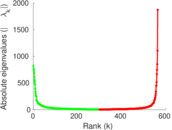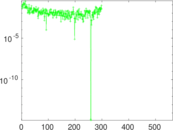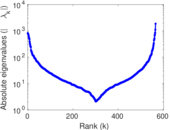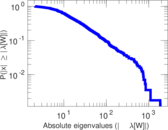A Song of Ice and Fire

This is the fictional social network of the series of fantasy novels "A Song of Ice and Fire" by George R. R. Martin (1996–present). An edge denotes that two characters are mentioned within fifteen words of each other; edge multiplicities denote the number of such appearances. This version of the dataset covers books one to five.

 Code IF Internal name asoiaf Name A Song of Ice and Fire Data source https://github.com/mathbeveridge/asoiaf AvailabilityDataset is available for download Consistency checkDataset passed all tests Category Miscellaneous network Dataset timestamp 1996 ⋯ 2011 Node meaning Character Edge meaning Co-appearance Network format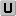Unipartite, undirected Edge typeUnweighted, multiple edges LoopsDoes not contain loops

Statistics

 Size n = 796 Volume m = 32,629 Unique edge count m̿ = 2,823 Loop count l = 0 Wedge count s = 81,158 Claw count z = 1,766,472 Cross count x = 37,318,726 Triangle count t = 5,655 Square count q = 78,782 4-Tour count T4 = 960,534 Maximum degree dmax = 122 Average degree d = 81.982 4 Fill p = 0.008 921 97 Average edge multiplicity m̃ = 11.558 3 Size of LCC N = 796 Diameter δ = 9 50-Percentile effective diameter δ0.5 = 2.848 72 90-Percentile effective diameter δ0.9 = 4.143 66 Median distance δM = 3 Mean distance δm = 3.410 82 Gini coefficient G = 0.788 981 Balanced inequality ratio P = 0.176 331 Relative edge distribution entropy Her = 0.886 437 Power law exponent γ = 1.779 17 Tail power law exponent γt = 2.321 00 Tail power law exponent with p γ3 = 2.321 00 p-value p = 0.051 000 0 Degree assortativity ρ = −0.115 391 Degree assortativity p-value pρ = 3.389 13 × 10−18 Clustering coefficient c = 0.209 037 Spectral norm α = 953.787 Algebraic connectivity a = 0.752 030 Spectral separation |λ1[A] / λ2[A]| = 1.717 35 Non-bipartivity bA = 0.578 642 Normalized non-bipartivity bN = 0.123 682 Algebraic non-bipartivity χ = 0.203 828 Spectral bipartite frustration bK = 0.007 184 18 Controllability C = 168 Relative controllability Cr = 0.211 055

Plots

Fruchterman–Reingold graph drawing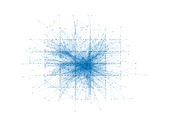Degree distribution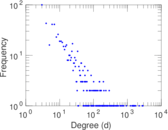Cumulative degree distribution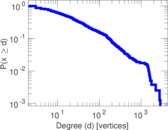Lorenz curve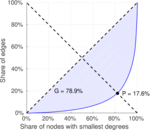Spectral distribution of the adjacency matrix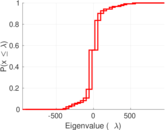Spectral distribution of the normalized adjacency matrix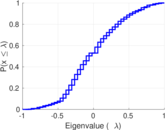Spectral distribution of the Laplacian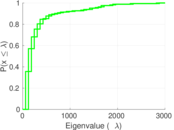Spectral graph drawing based on the adjacency matrix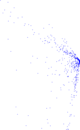Spectral graph drawing based on the Laplacian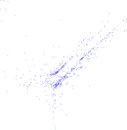Spectral graph drawing based on the normalized adjacency matrix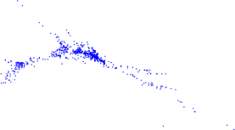Degree assortativity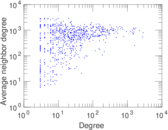Zipf plot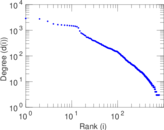Hop distribution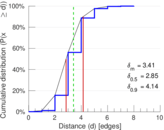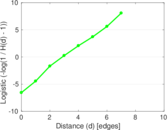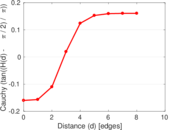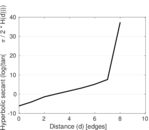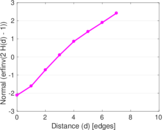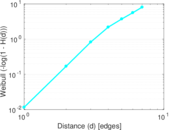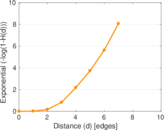Double Laplacian graph drawing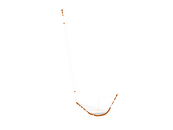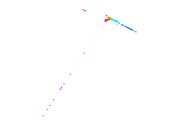Delaunay graph drawing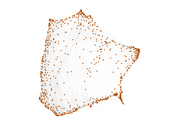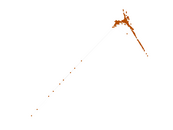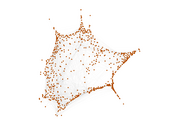Edge weight/multiplicity distribution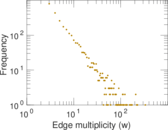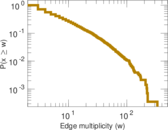Clustering coefficient distribution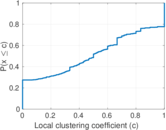Average neighbor degree distribution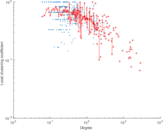SynGraphy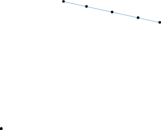Matrix decompositions plots